### 7.1 Synopsis

The aim of APESolver is to predict aerodynamic sound generation. Through the application of a splitting technique, the flow-induced acoustic field is totally decoupled from the underlying incompressible hydrodynamic field. The acoustic perturbation equations (APE-1/APE-4) proposed by Ewert and Shroeder are employed as the governing equations of the acoustic field and they assure stable aeroacoustic simulation due to the suppression of the term related to the production of perturbed vorticity. These equations are similar to the linearised perturbed compressible equations, but while in the original formulation the flow decomposition is based on solenoidal vortical perturbations as well as irrotational acoustic perturbations, in this case perturbations are assumed to be exclusively of acoustic nature.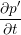+ c2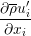+ c2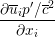= c2q c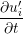+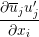+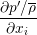= 0

where (ui,p,ρ,c2 = γpρ) represents the base flow and (u′i,p′) the perturbations. c2qc is the acoustic source term.